Publicité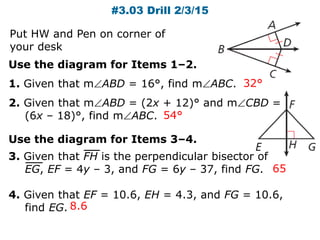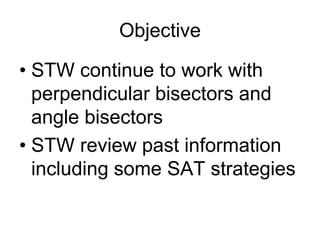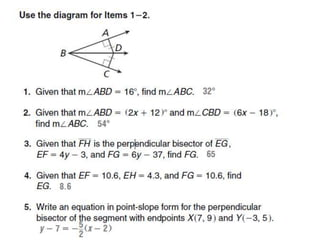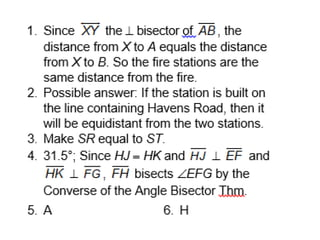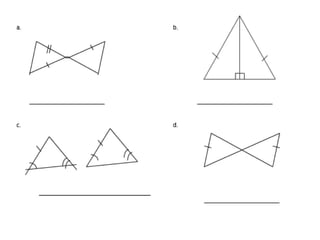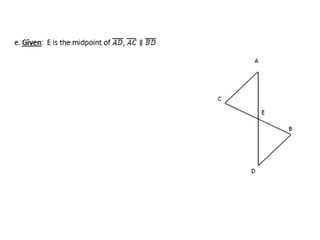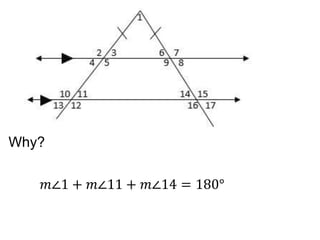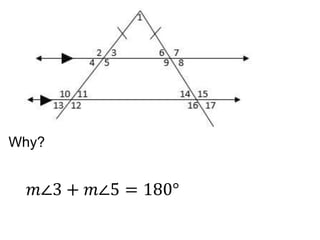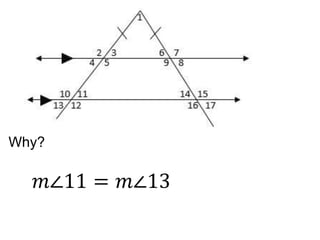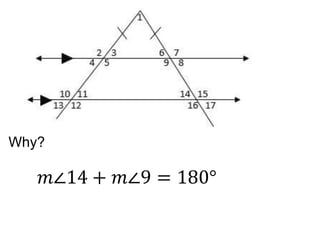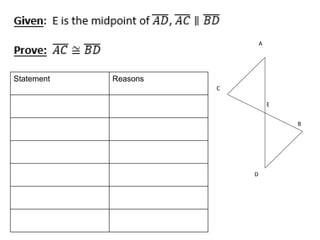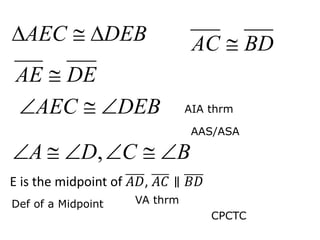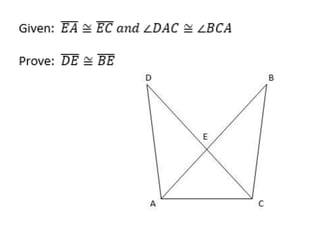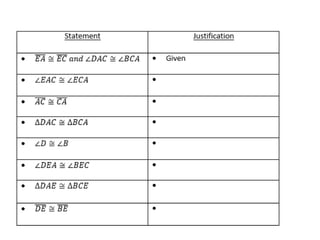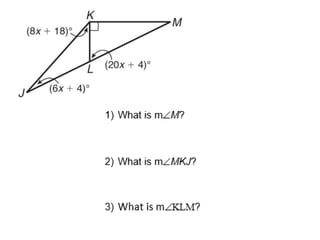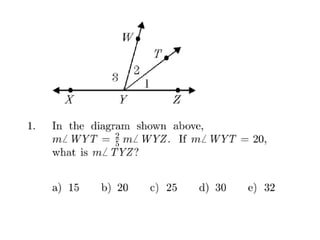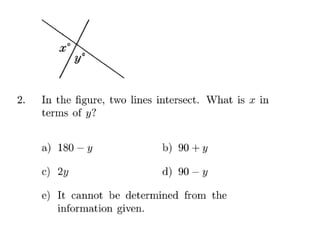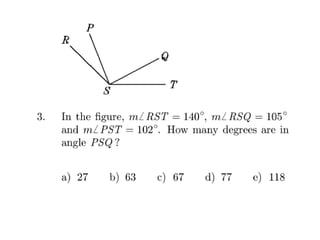1 sur 18
Publicité

### Chapter 5 unit f 003 review and more updated

1. #3.03 Drill 2/3/15 Use the diagram for Items 1–2. 1. Given that mABD = 16°, find mABC. 2. Given that mABD = (2x + 12)° and mCBD = (6x – 18)°, find mABC. 32° 54° 65 8.6 Use the diagram for Items 3–4. 3. Given that FH is the perpendicular bisector of EG, EF = 4y – 3, and FG = 6y – 37, find FG. 4. Given that EF = 10.6, EH = 4.3, and FG = 10.6, find EG. Put HW and Pen on corner of your desk
2. Objective • STW continue to work with perpendicular bisectors and angle bisectors • STW review past information including some SAT strategies
3. 𝑚∠1 + 𝑚∠11 + 𝑚∠14 = 180° Why?
4. Why? 𝑚∠3 + 𝑚∠5 = 180°
5. Why? 𝑚∠11 = 𝑚∠13
6. Why? 𝑚∠14 + 𝑚∠9 = 180°
7. Statement Reasons A B D C E
8. DEAE  DEBAEC  BCDA  , DEBAEC  BDAC  E is the midpoint of 𝐴𝐷, 𝐴𝐶 ∥ 𝐵𝐷 Def of a Midpoint VA thrm AIA thrm AAS/ASA CPCTC
Publicité